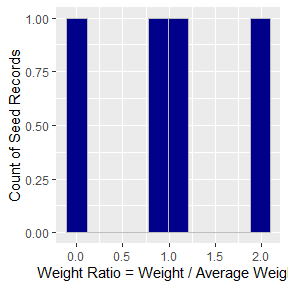Introduction

Iterative proportional fitting (IPF) is used in many disciplines to adjust an initial set of weights to match various marginal distributions. This package implements the iterative proportional updating algorithm based on the paper from Arizona State University (IPU). In survey raking or population synthesis, the IPU algorithm has the added advantage of being able to match household- and person-level marginal targets.

The primary functions of the ipfr package are discussed below.

• ipu_matrix()
• ipu()
• synthesize()
suppressPackageStartupMessages({
library(ipfr)
library(dplyr)
})

Example: 2D / Matrix

The first example shows how to use ipfr to solve one of the most common IPF problems: Given a matrix of starting weights and a set of row and column targets, balance the matrix in order to satisfy both row and column targets.

The resulting matrix satisfies both row and column targets while retaining a similar relative distribution of weights in each cell.

Example: Household survey (simple)

The matrix example is common and conceptually simple, but makes thinking about higher-dimension problems difficult. A much better way to think about the problem (whether in two or ten dimensions) is as a table.

This example creates a household survey that will act as the seed (like the matrix from the last example). The number of persons and autos for each household is listed in the size and autos columns. A starting weight of 1 is also included.

Assume that we know the total number of households by size and households by auto-ownership from the Census. These target marginal distributions are just like our row and column totals from the previous example. Targets are passed into the ipu function as shown below. Take the size targets, for example:

• The list name size corresponds to the size column in the survey.
• The column names 1 and 2 correspond to the unique values in the survey’s size column.

A call to ipu() returns a named list of results.

The first element is the resulting weight table. The primary seed has three columns appended:

• weight
• The new/expanded weight of the record.
• avg_weight
• The average weight, which is the total target / number of seed records.
• weight_factor
• Each record’s weight divided by the average weight. This is a useful diagnostic to measure how extreme a weight is.

The second element is a histogram of the weight_factor. This provides a quick overview of the distribution of weights. Many large or small weights can indicate underlying problems even if the ipu converged and matches targets. For instance, a record with a weight factor of 50 means that it is greatly over-represented in the re-weighted survey.The next element is a comparison back to the targets provided. With complex seed and target tables, this makes investigating results quick and easy.

If secondary targets are provided to ipu(), a fourth item in the list will contain a secondary_comp table.

Example: Add person targets

In household survey expansion, it is common to want to control for certain features that describe households (like size) while controlling for other attributes that describe people (like age). This is possible with the ipu() function.

This example is taken directly from the Arizona paper on page 20: http://citeseerx.ist.psu.edu/viewdoc/download?doi=10.1.1.537.723&rep=rep1&type=pdf

In this example, household type could represent size (e.g. 1-person and 2-person households). Person type could represent age groups (e.g. under 18, between 18 and 50, and over 50).

setup_arizona() creates the seed and target tables used in the example.

• The household seed table is the primary_seed
• The household target list is the primary_target
• The person seed table is the secondary_seed
• The person target list is the secondary_target

In the interest of keeping vignette build time short, the ipu() algorithm is only run for 30 iterations. After running for 400 or more iterations, the results match closely to those shown in the paper.

The comparison table for the secondary (person) marginals is now included in result. Feel free to run the code chunk above for 400 or more iterations and then look again.

Example 3: Using multiple geographies

ipu() allows different geographies to be specified for different marginal tables. There are a few rules that make this possible, but in short, a geography field in each target table tells the algorithm which scale to constrain to. If no geography field is present in a target table, it applies to the entire seed table (i.e. region).

All of the following rules are checked by the function and a warning message will show which (if any) is violated.

• The primary seed table must contain all geography fields used by any target tables.
• Do not duplicate geography fields on the secondary seed table.
• This prevents potential errors/inconsistencies between seed tables.
• All fields that designate geographies must start with “geo_” e.g.
• geo_tract
• geo_region
• geo_state

To demonstrate, the Arizona example is modified to add two different tracts for household controls but to still control the person targets at the regional level.

Run the IPU algorithm. Again, for vignette build time, only 30 iterations are performed. Run the code yourself with max_iterations set to 600 to see the converged result.

The tables below show the results compared back to targets.